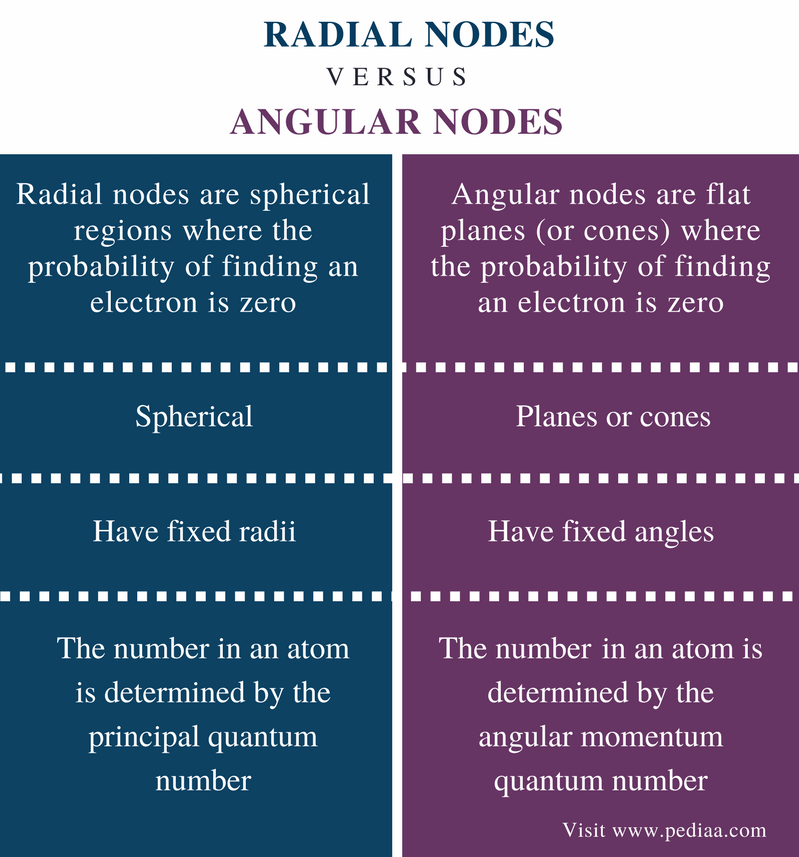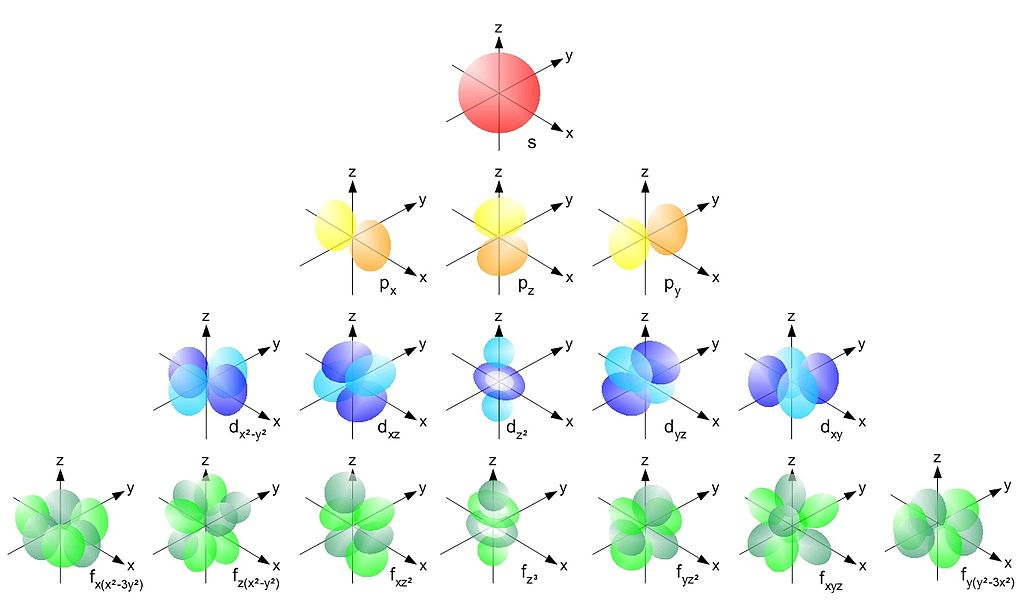# Difference Between Radial and Angular Nodes

## Main Difference – Radial vs Angular Nodes

An atomic orbital or electronic orbital is the region of an atom where an electron can be found with the highest probability. An atom contains protons and neutrons at the center of the atom, which is called the nucleus. There are no electrons in the nucleus. Electrons are dispersed around the nucleus. But these electrons are in movement around the nucleus in specific paths known as electron orbitals or electron shells. These electron shells are composed of subshells. Depending on the angular momentum quantum number, a subshell contains one or more orbitals: s orbital, p orbital, d orbital, and f orbital. These orbitals can be in different planes. Each orbital in a particular plane is known as a lobe. Electrons are found within these lobes. But there are planes where no electrons can be found. These are called nodes. There are two types of nodes as radial nodes and angular nodes. The main difference between radial nodes and angular nodes is that radial nodes are spherical whereas angular nodes are typically flat planes.

### Key Areas Covered

1. What are Lobes and Nodes
– Explanation of Lobes and Nodes
– Definition, Shape, and Determination
3. What are Angular Nodes
– Definition, Shape, and Determination
4. What are the Similarities Between Radial and Angular Nodes
– Outline of Common Features
5. What is the Difference Between Radial and Angular Nodes
– Comparison of Key Differences

Key Terms: Angular Node, Atom, Atomic Orbital, Electron, Electron Shell, Lobe, Node, Nucleus, Radial Node, Quantum Numbers## What are Lobes and Nodes

First and foremost, let us understand properly what a lobe is. As explained in the introduction, atoms are composed of protons, neutrons, and electrons. Protons and neutrons reside in the center of the atom, which is called the nucleus. But there are no electrons in the nucleus. Electrons are in a continuous movement around the nucleus. They do not move in random paths. There are specific paths where electrons can be located. These are known as electron shells. An electron shell is a region where an electron can reside with the highest probability.

Electrons shells are located at different distances from the nucleus. They have specific, discrete energies. Therefore, these electron shells are also known as energy levels. These are named as K, L, M, N, etc. starting from the nearest to the nucleus. The smallest electron shell has the lowest energy.

Each and every electron shell is characterized using quantum numbers. Electron shells have sub shells. These sub shells are composed of orbitals. These orbitals are different from each other based on the angular momentum of electrons in those orbitals. These orbitals have different shapes as well. The sub shells are named as s, p, d, and f.

Sub shells have lobes (orbitals) in different planes. Lobes are the regions where electrons reside. The size, shape and the number of these lobes are different from each other for different orbitals.Figure 1: Different Lobes of Orbitals

As shown in the above image, the lobes are located in different planes. The planes where no orbital can be seen are called nodes. There are no electrons in nodes. Therefore, nodes are the regions where a zero probability for an electron to be found. For example, as given in the above image, there are no orbitals for the planes dxz and dyz for the dxy orbital.

Radial nodes are spherical regions where the probability of finding an electron is zero. This sphere has a fixed radius. Therefore, radial nodes are determined radially. Radial nodes occur as the principal quantum number increases. The principal quantum number represents electron shells.

When finding radial nodes, the radial probability density function can be used. The radial probability density function gives the probability density for an electron to be at a point located the distance r from the proton. The following equation is used for this purpose.

Ψ(r,θ,Φ)   =  R(r) Y(θ,Φ)

Where Ψ is the wave function, R(r) is the radial component (depends upon only the distance from the nucleus) and Y(θ,φ) is the angular component. A radial node occurs when R(r) component becomes zero.

## What are Angular Nodes

Angular nodes are flat planes (or cones) where the probability of finding an electron is zero. This means we cannot ever find an electron in an angular (or any other) node. While radial nodes are located at fixed radii, angular nodes are located at fixed angles. The number of angular node present in an atom is determined by the angular momentum quantum number. Angular nodes occur as the angular momentum quantum number increases.

## Similarities Between Radial and Angular Nodes

• Both represent the regions in atoms where an electron cannot be found.
• Both types depend on quantum numbers.

## Difference Between Radial and Angular Nodes

### Definition

Radial Nodes: Radial nodes are spherical regions where the probability of finding an electron is zero.

Angular Nodes: Angular nodes are flat planes (or cones) where the probability of finding an electron is zero.

### Shape

Angular Nodes: Angular nodes are planes or cones.

### Characteristic Properties

Angular Nodes: Angular nodes have fixed angles.

### Number of Nodes

Radial Nodes: The number of radial nodes present in an atom is determined by the principal quantum number.

Angular Nodes: The number of angular nodes present in an atom is determined by the angular momentum quantum number.

### Conclusion

Nodes are regions in atoms where no electron can ever be found. There are two types of nodes as radial nodes and angular nodes. The main difference between radial nodes and angular nodes is that radial nodes are spherical whereas angular nodes are typically flat planes.

##### References:

1. “Radial Nodes.” Chemistry LibreTexts, Libretexts, 8 Jan. 2017, Available here.
2. “Electronic Orbitals.” Chemistry LibreTexts, Libretexts, 19 Nov. 2017, Available here.
3. “Atomic orbital.” Wikipedia, Wikimedia Foundation, 9 Dec. 2017, Available here.

##### Image Courtesy:

1. Single electron orbitals” By haade – Own work, based on various sources, sketch NOT computer generated models (CC BY-SA 3.0) via Commons Wikimedia